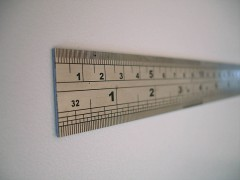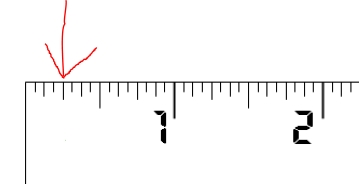# Where Is 1/4 Of A Inch On A 12 Inch Ruler?

## 7 Answers

Ray Hueston answeredWhen looking at the 12 inch ruler, finding a quarter of an inch may seem like a mission, but there’s no need to worry! Follow the ruler to the first inch and you will see that there are individual lines between zero and one. These are all a sixteenth of an inch, thus the fourth line that is slightly longer is a quarter of an inch! It isn’t as difficult as you may think.

You can then use the markings to work out half an inch, as well as three-quarters of an inch. Alternatively, if you notice that there is a thicker or longer line midway between zero and one, you can assume that this is half an inch, and then you can look to the longer line between the beginning of the ruler and the half an inch mark which will also give you a quarter measurement.

Once you have found this method easy, you can move on to becoming aware of where the measurement for an inch-and-a-half is, and so on and so forth. However, it can be daunting when you don’t know what all the lines between the main measurements are.

Do remember that you will be able to see numerical denominations printed onto the ruler in some cases, and other instruments such as a tape measure can allow you to accurately measure the length of items (as well as distances) with clearly-marked inches along the edges for you to reference with ease. Practice makes perfect, and for those of us accustomed to centimetres, it just takes a while to get into the swing of things.

thanked the writer.
Anonymous answered
View the pic please - this is a close up on a 2 inch segment.thanked the writer.
Anonymous answered
Count from zero, 1, 2, 3, 4, then there is 1/4 inch.
thanked the writer.
Mr. Adamo answered
At the beginning of zero ( 0 ) heading toward one ( 1 ) inch there are three small lines (1/16" of an inch each) and then a longer line for the fourth line. This line is 1/4" (one quarter) of an inch. All inches have sixteen lines adding up to within that individual inch. Thus if you look for a quarter of an inch you have four ( 4 ) lines, and then a half of an inch is going to be eight ( 8 ) lines, and so, how much would three quarters ( 3/4" ) of inch be? That means twelve ( 12 ) out of the final sixteen inches. Easy.
thanked the writer.
Sally answered
Count over 4 hash marks and that's 1/4".
thanked the writer.
Anonymous answered
It is on the fourth hash mark.
thanked the writer.
Charles Ruiz answered

the " 12 inch ruler" part is meaningless because 1/4 inch is an absolute no matter where you masure it on a ruler, a yardstick,a mile,, amillion miles....the moon...mars..pluto...

thanked the writer.

## Answer Question